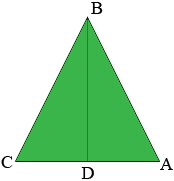SEARCH HOMEMath Central Quandaries & QueriesQuestion from Evelyn, a student: Hi! I am facing problem finding the height of the isosceles triangle. I have found one of the question that tell about how to do it. However I am still facing problem as I am not fully understand by it. I am thinking of finding the height by using the area of trapezium. But the area of trapezium is not given as a clue. And I don't have an idea of how to find the height. Please help me. ThanksHi Evelyn,

Suppose the triangle is ABC and |AB| = |BC|. Draw a line from D, the midpoint of CA to B.Since triangles BCD and ABD are congruent, angle BDA is a right angle and |BD| is the height of the triangle. You know |AB| and |DA| = 1/2 |CA| and triangle BDA is a right triangle so you can use Pythagoras theorem to find |BD|.

PennyMath Central is supported by the University of Regina and The Pacific Institute for the Mathematical Sciences.